# Test: Combinational Circuit- 2

## 15 Questions MCQ Test Question Bank for GATE Computer Science Engineering | Test: Combinational Circuit- 2

Description
Attempt Test: Combinational Circuit- 2 | 15 questions in 45 minutes | Mock test for Computer Science Engineering (CSE) preparation | Free important questions MCQ to study Question Bank for GATE Computer Science Engineering for Computer Science Engineering (CSE) Exam | Download free PDF with solutions
QUESTION: 1

### The following circuit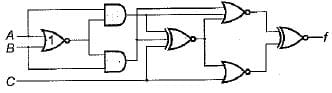Can be represented as:

Solution:

From the given diagram we can see that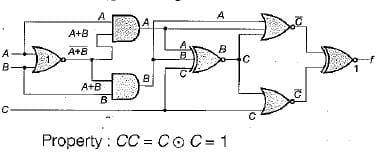QUESTION: 2

### The circuit shown below converts.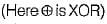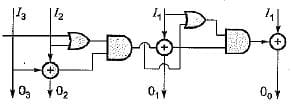Solution:

if input is 1010 it generate 1101 which is same as gray to binary code converter.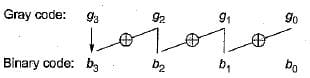QUESTION: 3

### The output of the circuit shown in following figure is equal to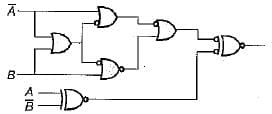Solution: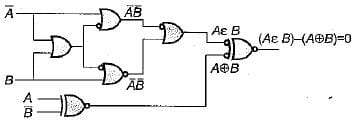Hence (b) is the correct option.

QUESTION: 4

The circuit given in figure is to be used to implement the function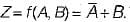What is the values should be selected I and J?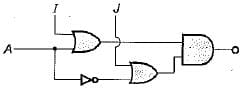Solution: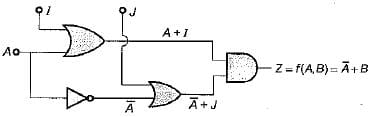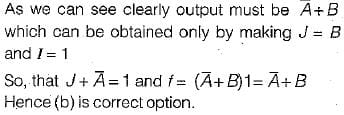QUESTION: 5

Minimum number of NAND gates required to implement sum in half-adder circuit is:

Solution: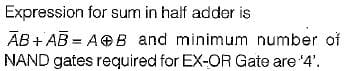QUESTION: 6

The following circuit is an implemented of: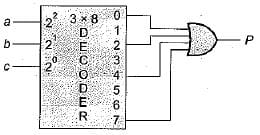3. Difference of full subtracte

Solution:

From the given circuit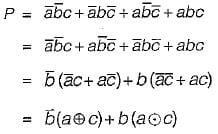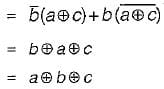For which only 1 and 3 satisfy. Hence correct option is (d),

QUESTION: 7

The circuit below represents function X{A, B, C, D) as: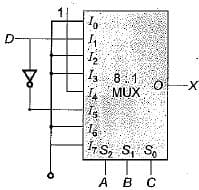Solution:

The given circuit represents the implementation of four variable function using 8: 1 MUX here. D as taken as the fourth i/p and A, B, C act as select lines.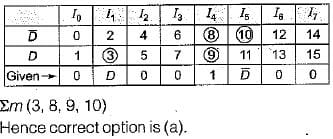QUESTION: 8

If half adders and full adders are implemented using gates, then for the addition of two 17 bit numbers (using minimum gates) the number of half adders and full adders required will be

Solution:

As we know that n bit full adder circuit can represent (n + 1) bits sum. In order to represent addition of two 17 bits numbers we require minimum of 16 full adder and 1 half adder.
Note: For the first bit we can use either HA or FA. Hence, for addition of two 17 bit numbers inspire of 17 full adders we can perform the same task using 16 FA and 1 HA.
Hence (c) is the correct option.

QUESTION: 9

To realize following function 'f'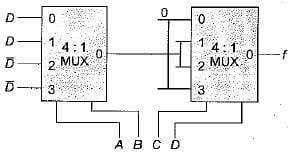How many minimum number of 2 input NAND gates are required

Solution: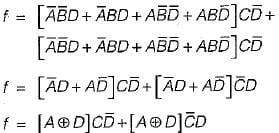So it required 10 NAND gates.

QUESTION: 10

Minimum number of 2 x 1 multiplexers required to realize the following function,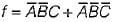Assume that inputs are available only in true forr and Boolean constants.1 and 0 are available.

Solution: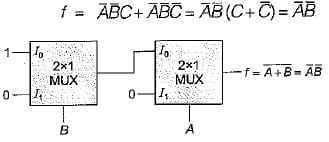QUESTION: 11

The number of full and half-adder required to add 16-bit numbers is

Solution:

To 16 bits number, 1 half and 15 full address or 16 full address are required.

QUESTION: 12

How many 3-to-8 line decoders with an enable input are needed to construct a 6-to-64 line decoder without using any other logic gates?

Solution: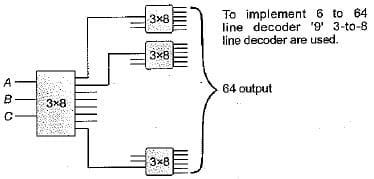QUESTION: 13

The following circuit implements a two-input AND gate using two 2-1 multiplexers.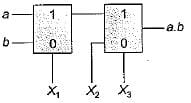What are the values of X1 X2, X3?

Solution: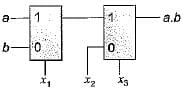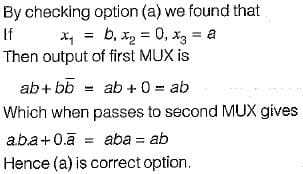QUESTION: 14

Consider excess-3 code that is used to represent integers 0 through 9 as shown below: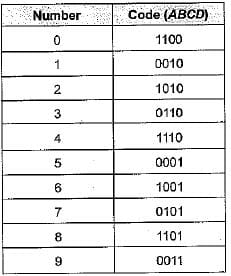Which of the following expressions is the correct one for an invalid code?

Solution:

Invalid code words are 0, 4, 4, 8, 11, 5.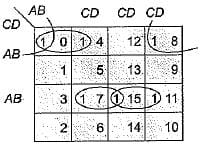So code will be a'c'd' + b'c'd' + bed + acd.

QUESTION: 15

What logic function is performed by the circuit shown below: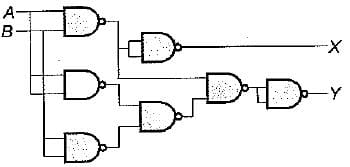Solution: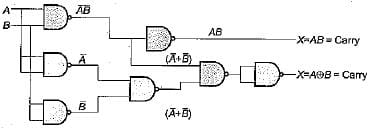Hence the given circuit represents Half adder.Use Code STAYHOME200 and get INR 200 additional OFF Use Coupon Code